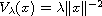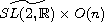Electron. J. Diff. Equ., Vol. 2013 (2013), No. 154, pp. 1-16.

### Global representations of the Heat and Schrodinger equation with singular potential Jose A. Franco, Mark R. Sepanski

Abstract:
The n-dimensional Schrodinger equation with a singular potentialis studied. Its solution space is studied as a global representation of. A special subspace of solutions for which the action globalizes is constructed via nonstandard induction outside the semisimple category. The space of K-finite vectors is calculated, obtaining conditions forso that this space is non-empty. The direct sum of solution spaces over such admissible values ofis studied as a representation of the (2n+1)-dimensional Heisenberg group.

Submitted February 28, 2013. Published July 2, 2013.
Math Subject Classifications: 22E70, 35Q41.
Key Words: Schr\"{o}dinger equation; heat equation; singular potential; Lie theory; \hfill\break\indent representation theory; globalization

Show me the PDF file (359 KB), TEX file, and other files for this article.Jose A. Franco University of North Florida 1 UNF Drive, Jacksonville, FL 32082, USA email: jose.franco@unf.edu Mark R. Sepanski Baylor University, One Bear Place # 97328 Waco, TX 76798, USA email: mark_sepanski@baylor.edu